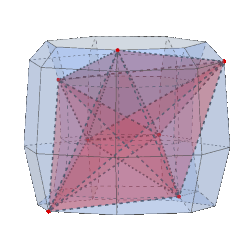Acronym ... Name general 7-2-stepprism© Circumradius sqrt(A2+B2) Coordinates (A sin(2πk/7), A cos(2πk/7), B sin(2πk/7), B cos(2πk/7))     0 ≤ k ≤ 6 Confer general polytopal classes: isogonal ExternallinksAn n-m-step prism is inscribed within an according variant of an n-duoprism, in fact it cycles a subset of its vertices in the n×n grid by means of an inclined line of slope n/m. It then uses the convex hull of that subset of points.

Let y = x(7,2) = sin(2π/7)/sin(π/7) = 1.801938 and z = x(7,3) = sin(3π/7)/sin(π/7) = 2.246980 then the edge sizes, as given below, would be a = sqrt(A2+y2B2), b = sqrt(y2A2+z2B2), and c = sqrt(z2A2+B2).

Incidence matrix

7 | 2 2 2 | 3 3 6  | 4 4  verf: bi-apiculated tet
--+-------+--------+----
2 | 7 * * | 2 1 2  | 3 2  a (5-valence)
2 | * 7 * | 1 0 2  | 2 1  b (3-valence)
2 | * * 7 | 0 2 2  | 1 3  c (4-valence)
--+-------+--------+----
3 | 2 1 0 | 7 * *  | 2 0  aab
3 | 1 0 2 | * 7 *  | 0 2  acc
3 | 1 1 1 | * * 14 | 1 1  abc
--+-------+--------+----
4 | 3 2 1 | 2 0 2  | 7 *  phyllic disphenoid type 1
4 | 2 1 3 | 0 2 2  | * 7  phyllic disphenoid type 2